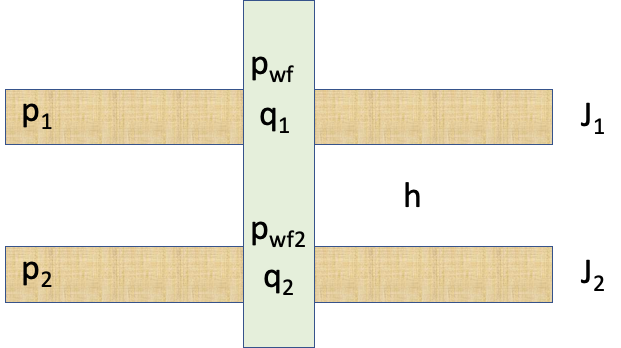# Dual-layer formation pressureFig. 1. Dual-layer well schematic

 (1)
 (2)
 (3)
 (4)

where

Well

$//$

total subsurface flowrate of the well

$//$

total well productivity Index

$//$

apparent formation pressure of dual-layer formation
Layer #1

$//$

bottom-hole pressure at Layer #1 top

$//$

total subsurface flowrate of the Layer #1

$//$

formation pressure of the Layer #1

$//$

productivity Index of the Layer #1
Layer #2

$//$

bottom-hole pr4essure at Layer #2 top

$//$

wellbore pressure loss between the tips of two layers

$//$

total subsurface flowrate of the Layer #2

$//$

formation pressure of the Layer #2

$//$

productivity Index of the Layer #2

In many practical cases one can safely assume:

 (5)

where

$//$ wellbore fuid density gravity constant true vertical height between $//$-th layer and reference layer $//$

The above equations are valid for both producers $//0\end{array} //]]>$ and injectors $//$.

 (6)
 (7)

 (8)
 (9)

and

 (10)
 (11)

or

 (12)